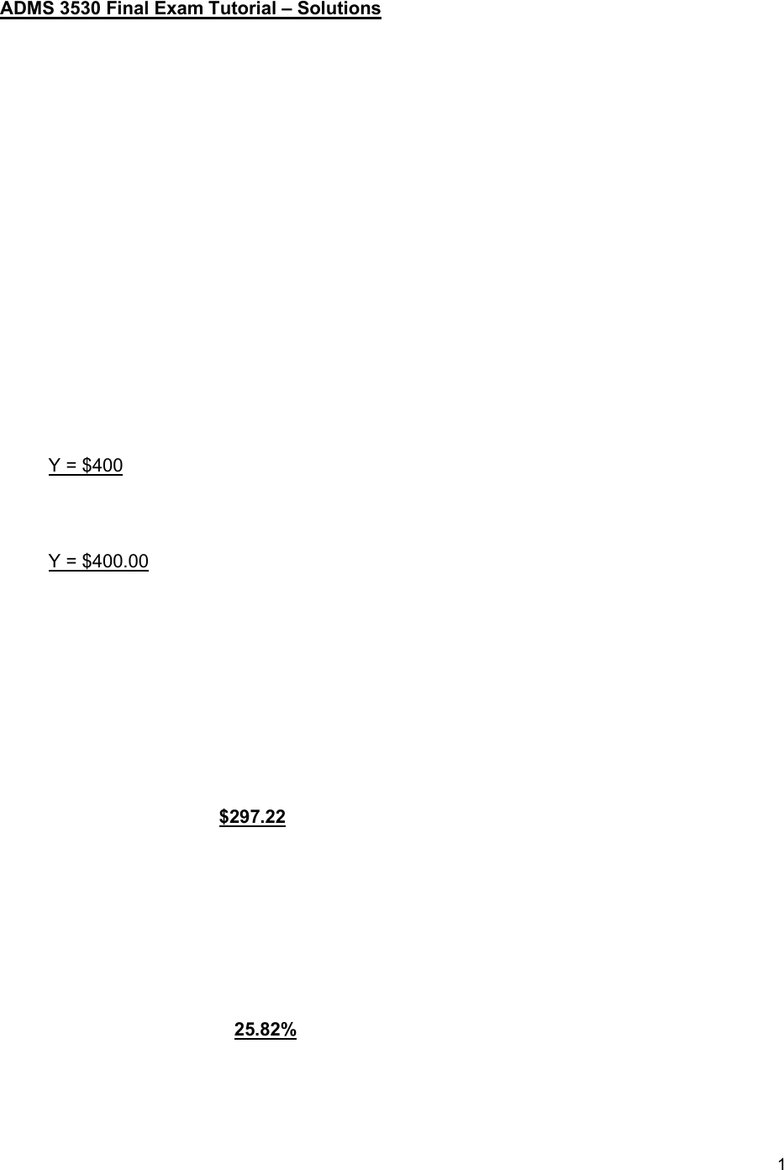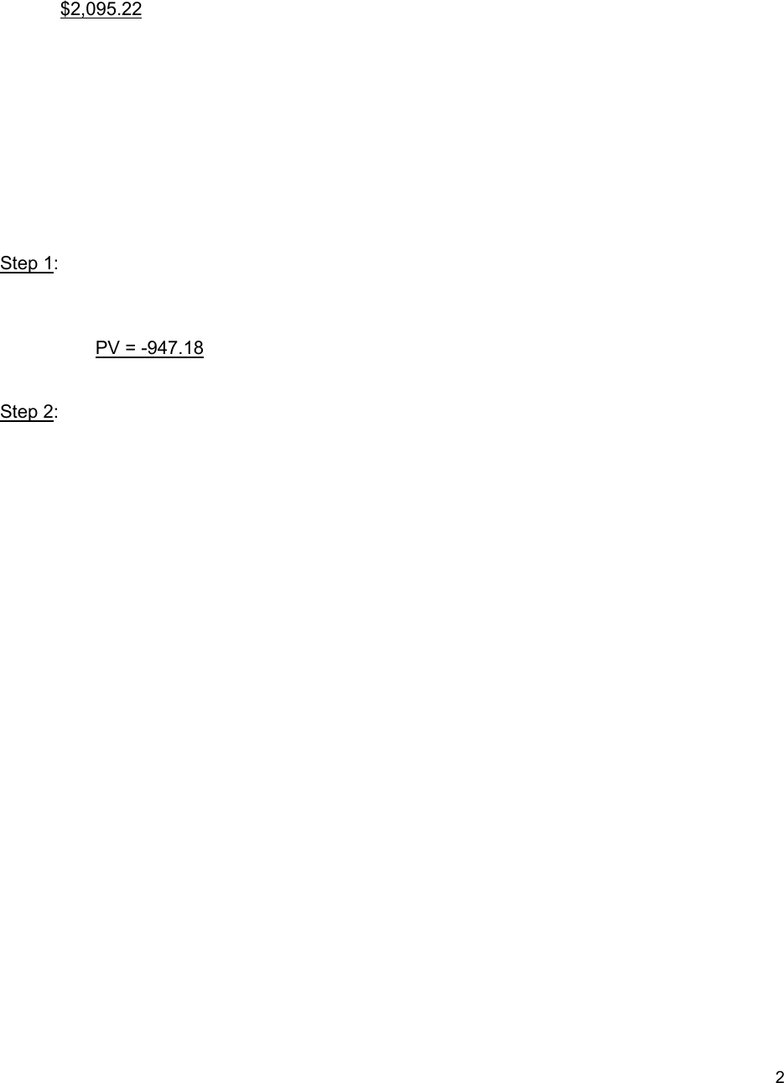Study Guides (400,000)
CA (160,000)
York (10,000)
Final

# ADMS 3530 Final: 3530_Final_Tutorial Solutions.SU19 (1)Exam

Department
Course Code
Professor
Alagurajah
Study Guide
Final

This preview shows pages 1-2. to view the full 7 pages of the document.Document1
1
ADMS 3530 Final Exam Tutorial Solutions
Example 1: Multiple Cash Flows
Detailed solution:
0 1 2 r = 4%
|-----------|-------------I FV2 = \$3032.32
\$200 “Y” 2400
You can use either
Option a) FV formula and bring all cash flows to t=2 or
Option b) PV formula and bring all cash flows to t=0
Option a) Bring all cash flows to t=2 (use FV or compound)
200(1 + r)2 + Y(1 + r)1 + 2400 = 3032.32
200(1.04)2 + Y (1.04) + 2400 = 3032.32
216.32 + Y(1.04) + 2400 = 3032.32
Y(1.04) = 3032.32 2400 216.32
Y(1.04) = 416
Y = 416 / (1.04)
Y = \$400
Option b) Bring all cash flows to t=0 (use PV and discount)
200 + Y/(1 + r)1 + 2400/(1 + r)2 = 3032.32/ (1 + r )2
Y = \$400.00
Example 2: Delayed Annuity
PMT = \$100, I = 9, n= 4, Fv =0, COMP PV
PV = \$323.97 at t=1 !!
Bring PV1 to PV0
PV0 = \$323.97 / (1.09)1 = \$297.22
Example 3: EAR
Using your calculator: PMT = \$1883.33, n = 12, PV = \$20,000, FV=0, Comp “i”
i= 1.9322%
EAR = (1+.019322)12 -1 = 25.82%
Example 4: Mortgages

Only pages 1-2 are available for preview. Some parts have been intentionally blurred.Document1
2
The monthly interest rate is given by:
(1 + im )12 = 1 + EAR = (1 + 3125%)² = 1.076406, that is im = 0.5142%
The monthly payment for the 20-year loan
320,000 = PMT x PVIFA(0.5142%,300)
PMT = \$2,095.22
Mortgage remaining at the end of 5 years 240 months remaining:
PMt = \$2095.22, n=240, i=.5142, Fv=0, COMP PV
PV = 288,480.10
Example 5: Bonds
5A) Solution: B) 7.39%
Current Yield = Annual Coupon Payment/ Bond Price
Step 1: Find price of bond today (7 years remaining)
PMT = 70/2 = 35.00, n = 7 x 2 = 14, FV = 1000, i = 8/2 = 4%, COMP PV
PV = -947.18
-> Current Yield = \$70/\$947.18 = 7.39%
Step 2: Find rate of return over holding period (3 years)
5B) Solution B) 6.3309%
4-year Rate of Return = [30 x FVIFA(2%,8) + 1,040 1,015] / 1,015 = 27.8314% The annual
rate of return is (1+27.8314%)1/4 1 = 6.3309%
5C) Solution: E)12.26%
FV 1,100
PMT 86
PV -925
N 5
CPT I/Y = 12.26
YTC = 12.26%